# Dynamic Pricing for Personal Unsecured Loans

Feb. 22, 2021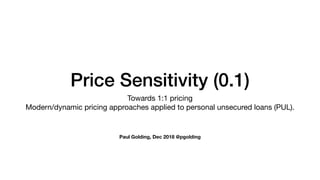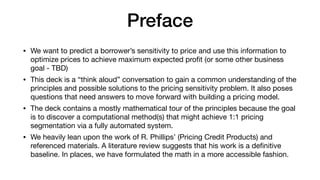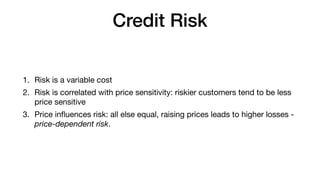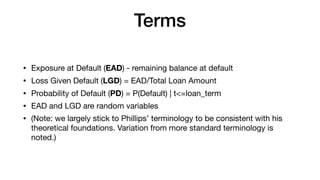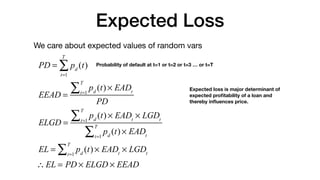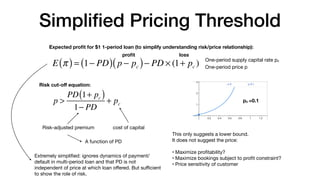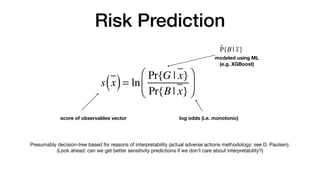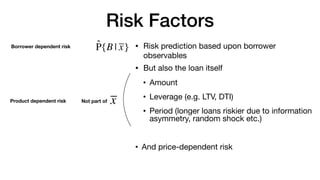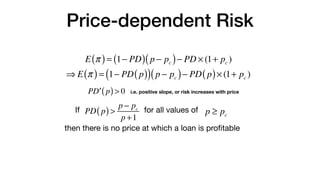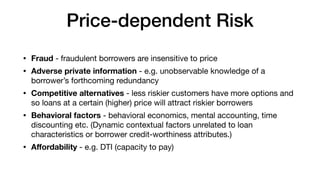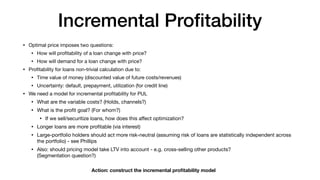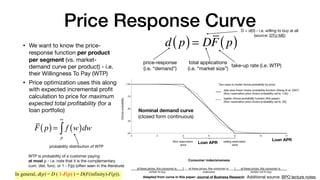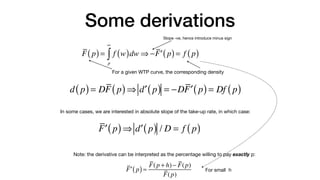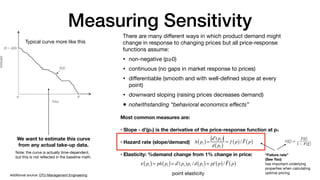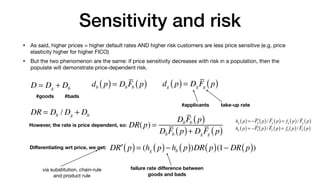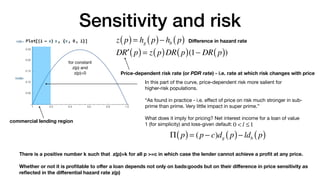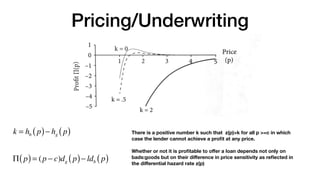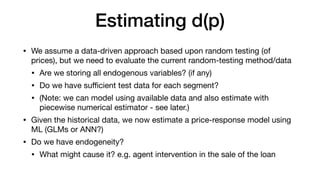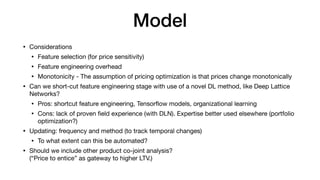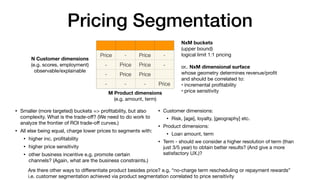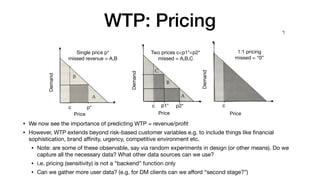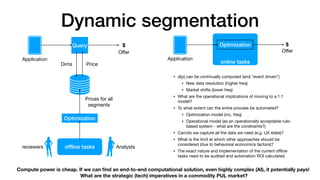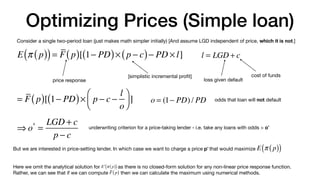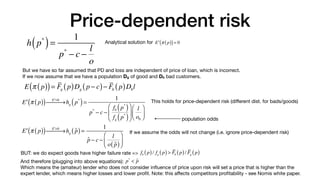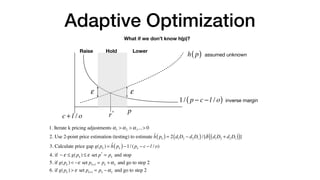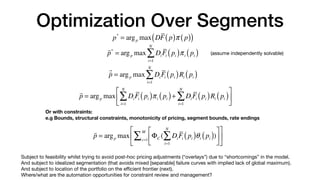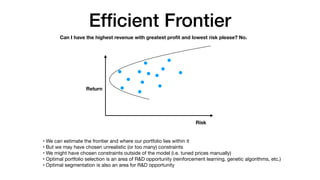1 of 27

### Dynamic Pricing for Personal Unsecured Loans

• 1. Price Sensitivity (0.1) Towards 1:1 pricing Modern/dynamic pricing approaches applied to personal unsecured loans (PUL). Paul Golding, Dec 2018 @pgolding
• 2. Preface • We want to predict a borrower’s sensitivity to price and use this information to optimize prices to achieve maximum expected profit (or some other business goal - TBD) • This deck is a “think aloud” conversation to gain a common understanding of the principles and possible solutions to the pricing sensitivity problem. It also poses questions that need answers to move forward with building a pricing model. • The deck contains a mostly mathematical tour of the principles because the goal is to discover a computational method(s) that might achieve 1:1 pricing segmentation via a fully automated system. • We heavily lean upon the work of R. Phillips’ (Pricing Credit Products) and referenced materials. A literature review suggests that his work is a definitive baseline. In places, we have formulated the math in a more accessible fashion.
• 3. Credit Risk 1. Risk is a variable cost 2. Risk is correlated with price sensitivity: riskier customers tend to be less price sensitive 3. Price influences risk: all else equal, raising prices leads to higher losses - price-dependent risk.
• 4. Terms • Exposure at Default (EAD) - remaining balance at default • Loss Given Default (LGD) = EAD/Total Loan Amount • Probability of Default (PD) = P(Default) | t<=loan_term • EAD and LGD are random variables • (Note: we largely stick to Phillips’ terminology to be consistent with his theoretical foundations. Variation from more standard terminology is noted.)
• 5. Expected Loss We care about expected values of random vars PD = pd (t) t=1 T ∑ EEAD = pd (t) × EADt t=1 T ∑ PD ELGD = pd (t) × EADt t=1 T ∑ × LGDt pd (t) × EADt t=1 T ∑ EL = pd (t) × EADt t=1 T ∑ × LGDt ∴ EL = PD × ELGD × EEAD Probability of default at t=1 or t=2 or t=3 … or t=T Expected loss is major determinant of expected profitability of a loan and thereby influences price.
• 6. Simplified Pricing Threshold E π ( )= 1− PD ( ) p − pc ( )− PD × (1+ pc ) One-period supply capital rate pc One-period price p Expected profit for \$1 1-period loan (to simplify understanding risk/price relationship): profit loss p > PD 1+ pc ( ) 1− PD + pc cost of capital Risk-adjusted premium This only suggests a lower bound. It does not suggest the price: • Maximize profitability? • Maximize bookings subject to profit constraint? • Price sensitivity of customer Extremely simplified: ignores dynamics of payment/ default in multi-period loan and that PD is not independent of price at which loan oﬀered. But suﬃcient to show the role of risk. A function of PD pc =0.1 Risk cut-oﬀ equation:
• 7. Risk Prediction s x ( )= ln Pr{G | x} Pr{B | x} ⎛ ⎝ ⎜ ⎞ ⎠ ⎟ score of observables vector log odds (i.e. monotonic) modeled using ML (e.g. XGBoost) Presumably decision-tree based for reasons of interpretability (actual adverse actions methodology: see D. Paulsen). (Look ahead: can we get better sensitivity predictions if we don’t care about interpretability?) P̂{B | x}
• 8. Risk Factors P̂{B | x} • Risk prediction based upon borrower observables • But also the loan itself • Amount • Leverage (e.g. LTV, DTI) • Period (longer loans riskier due to information asymmetry, random shock etc.) • And price-dependent risk Not part of x Borrower dependent risk Product dependent risk
• 9. Price-dependent Risk If for all values of E π ( )= 1− PD ( ) p − pc ( )− PD × (1+ pc ) ⇒ E π ( )= 1− PD p ( ) ( ) p − pc ( )− PD p ( )× (1+ pc ) P ′ D p ( )> 0 i.e. positive slope, or risk increases with price PD p ( )> p − pc p +1 p ≥ pc then there is no price at which a loan is profitable
• 10. Price-dependent Risk • Fraud - fraudulent borrowers are insensitive to price • Adverse private information - e.g. unobservable knowledge of a borrower’s forthcoming redundancy • Competitive alternatives - less riskier customers have more options and so loans at a certain (higher) price will attract riskier borrowers • Behavioral factors - behavioral economics, mental accounting, time discounting etc. (Dynamic contextual factors unrelated to loan characteristics or borrower credit-worthiness attributes.) • Aﬀordability - e.g. DTI (capacity to pay)
• 11. Incremental Profitability • Optimal price imposes two questions: • How will profitability of a loan change with price? • How will demand for a loan change with price? • Profitability for loans non-trivial calculation due to: • Time value of money (discounted value of future costs/revenues) • Uncertainty: default, prepayment, utilization (for credit line) • We need a model for incremental profitability for PUL • What are the variable costs? (Holds, channels?) • What is the profit goal? (For whom?) • If we sell/securitize loans, how does this aﬀect optimization? • Longer loans are more profitable (via interest) • Large-portfolio holders should act more risk-neutral (assuming risk of loans are statistically independent across the portfolio) - see Phillips • Also: should pricing model take LTV into account - e.g. cross-selling other products?   (Segmentation question?) Action: construct the incremental profitability model
• 12. Price Response Curve • We want to know the price- response function per product per segment (vs. market- demand curve per product) - i.e. their Willingness To Pay (WTP) • Price optimization uses this along with expected incremental profit calculation to price for maximum expected total profitability (for a loan portfolio) d p ( )= DF p ( ) total applications (i.e. “market size”) take-up rate (i.e. WTP) price-response (i.e. “demand”) Adapted from curve in this paper: Journal of Business Research Loan APR Loan APR F p ( )= f w ( )dw p ∞ ∫ probability distribution of WTP WTP is probability of a customer paying at most p - i.e. note that it is the complementary cum. dist. func. or 1 - F(p) (often seen in the literature) D = d(0) - i.e. willing to buy at all (source: DTU ME) Additional source: BPO lecture notes. Nominal demand curve (closed form continuous) In general, d(p) = D ( 1-F(p) ) = D(F(infinity)-F(p)).
• 13. Some derivations d p ( )= DF p ( )⇒ ′ d p ( ) = −D ′ F p ( )= Df p ( ) F p ( )= f w ( )dw p ∞ ∫ ⇒ − ′ F p ( )= f p ( ) For a given WTP curve, the corresponding density Slope -ve, hence introduce minus sign In some cases, we are interested in absolute slope of the take-up rate, in which case: ′ F p ( )⇒ ′ d p ( ) / D = f p ( ) Note: the derivative can be interpreted as the percentage willing to pay exactly p: ′ F p ( )≈ F(p + h)− F(p) F(p) For small h
• 14. Measuring Sensitivity Typical curve more like this Note: the curve is actually time-dependent, but this is not reflected in the baseline math. There are many diﬀerent ways in which product demand might change in response to changing prices but all price-response functions assume: • non-negative (p≥0) • continuous (no gaps in market response to prices) • diﬀerentiable (smooth and with well-defined slope at every point) • downward sloping (raising prices decreases demand) • notwithstanding “behavioral economics eﬀects” Additional source: DTU Management Engineering Most common measures are: • Slope - d’(p1) is the derivative of the price-response function at p1 • Hazard rate (slope/demand) • Elasticity: %demand change from 1% change in price: ε p1 ( )= ph p1 ( )= ′ d (p1)p1 / d p1 ( )= pf p ( )/ F p ( ) h p1 ( )= ′ d (p1) d p1 ( ) = f p ( )/ F p ( ) point elasticity We want to estimate this curve from any actual take-up data. “Failure rate” (See Yao) has important underlying properties when calculating optimal pricing.
• 15. Sensitivity and risk • As said, higher prices = higher default rates AND higher risk customers are less price sensitive (e.g. price elasticity higher for higher FICO) • But the two phenomenon are the same: if price sensitivity decreases with risk in a population, then the populate will demonstrate price-dependent risk. db p ( )= DbFb p ( ) D = Dg + Db dg p ( )= DgFg p ( ) #applicants take-up rate #goods #bads DR = Db / Dg + Db However, the rate is price dependent, so: DR(p) = DbFb p ( ) DbFb p ( )+ DgFg p ( ) Diﬀerentiating wrt price, we get: D ′ R p ( )= (hg p ( )− hb p ( ))DR p ( )(1− DR p ( )) failure rate diﬀerence between goods and bads hg p ( )= − ′ Fg p ( )/ Fg p ( )= fg p ( )/ Fg p ( ) hb p ( )= − ′ Fb p ( )/ Fb p ( )= fb p ( )/ Fb p ( ) via substitution, chain-rule and product rule
• 16. Sensitivity and risk D ′ R p ( )= z p ( )DR p ( )(1− DR p ( )) z p ( )= hg p ( )− hb p ( ) Diﬀerence in hazard rate Price-dependent risk rate (or PDR rate) - i.e. rate at which risk changes with price commercial lending region for constant z(p) and z(p)>0 In this part of the curve, price-dependent risk more salient for higher-risk populations. “As found in practice - i.e. eﬀect of price on risk much stronger in sub- prime than prime. Very little impact in super prime.” What does it imply for pricing? Net interest income for a loan of value 1 (for simplicity) and loss-given default: Π p ( )= (p − c)dg p ( )− ldb p ( ) 0 < l ≤1 There is a positive number k such that z(p)>k for all p >=c in which case the lender cannot achieve a profit at any price. Whether or not it is profitable to oﬀer a loan depends not only on bads:goods but on their diﬀerence in price sensitivity as reflected in the diﬀerential hazard rate z(p)
• 17. Pricing/Underwriting Π p ( )= (p − c)dg p ( )− ldb p ( ) There is a positive number k such that z(p)>k for all p >=c in which case the lender cannot achieve a profit at any price. Whether or not it is profitable to oﬀer a loan depends not only on bads:goods but on their diﬀerence in price sensitivity as reflected in the diﬀerential hazard rate z(p) k = hb p ( )− hg p ( )
• 18. Estimating d(p) • We assume a data-driven approach based upon random testing (of prices), but we need to evaluate the current random-testing method/data • Are we storing all endogenous variables? (if any) • Do we have suﬃcient test data for each segment? • (Note: we can model using available data and also estimate with piecewise numerical estimator - see later.) • Given the historical data, we now estimate a price-response model using ML (GLMs or ANN?) • Do we have endogeneity? • What might cause it? e.g. agent intervention in the sale of the loan
• 19. Model • Considerations • Feature selection (for price sensitivity) • Feature engineering overhead • Monotonicity - The assumption of pricing optimization is that prices change monotonically • Can we short-cut feature engineering stage with use of a novel DL method, like Deep Lattice Networks? • Pros: shortcut feature engineering, Tensorflow models, organizational learning • Cons: lack of proven field experience (with DLN). Expertise better used elsewhere (portfolio optimization?) • Updating: frequency and method (to track temporal changes) • To what extent can this be automated? • Should we include other product co-joint analysis?   (“Price to entice” as gateway to higher LTV.)
• 20. Pricing Segmentation M Product dimensions (e.g. amount, term) N Customer dimensions (e.g. scores, employment) observable/explainable Price - Price - - Price Price - - Price Price - - - Price NxM buckets (upper bound) logical limit 1:1 pricing or.. NxM dimensional surface whose geometry determines revenue/profit and should be correlated to: • incremental profitability • price sensitivity • Smaller (more targeted) buckets => profitability, but also complexity. What is the trade-oﬀ? (We need to do work to analyze the frontier of ROI trade-oﬀ curves.) • All else being equal, charge lower prices to segments with: • higher inc. profitability • higher price sensitivity • other business incentive e.g. promote certain channels? (Again, what are the business constraints.) • Customer dimensions: • Risk, [age], loyalty, [geography] etc. • Product dimensions: • Loan amount, term • Term - should we consider a higher resolution of term (than just 3/5 year) to obtain better results? (And give a more satisfactory UX.)? Are there other ways to diﬀerentiate product besides price? e.g. “no-charge term rescheduling or repayment rewards” i.e. customer segmentation achieved via product segmentation correlated to price sensitivity
• 21. WTP: Pricing Demand Price Demand Price Demand Price p* p1* p2* c c c Single price p* missed revenue = A,B Two prices c<p1*<p2* missed = A,B,C 1:1 pricing missed = “0” • We now see the importance of predicting WTP = revenue/profit • However, WTP extends beyond risk-based customer variables e.g. to include things like financial sophistication, brand aﬃnity, urgency, competitive environment etc. • Note: are some of these observable, say via random experiments in design (or other means). Do we capture all the necessary data? What other data sources can we use? • i.e. pricing (sensitivity) is not a “backend” function only • Can we gather more user data? (e.g. for DM clients can we aﬀord “second stage?”)
• 22. online tasks Dynamic segmentation Query \$ Price Dims Optimization Prices for all segments offline tasks Optimization \$ Oﬀer Oﬀer Application Application Analysts reviewers • d(p) can be continually computed (and “event driven”) • New data resolution (higher freq) • Market shifts (lower freq) • What are the operational implications of moving to a 1:1 model? • To what extent can the entire process be automated? • Optimization model (inc. freq) • Operational model (as an operationally acceptable rule- based system - what are the constraints?) • Can/do we capture all the data we need (e.g. UX state)? • What is the limit at which other approaches should be considered (due to behavioral economics factors)? • The exact nature and implementation of the current oﬄine tasks need to be audited and automation ROI calculated Compute power is cheap. If we can find an end-to-end computational solution, even highly complex (AI), it potentially pays! What are the strategic (tech) imperatives in a commodity PUL market?
• 23. Optimizing Prices (Simple loan) E π p ( ) ( )= F p ( )[ 1− PD ( )× p − c ( )− PD × l] Consider a single two-period loan (just makes math simpler initially) [And assume LGD independent of price, which it is not.] l = LGD + c [simplistic incremental profit] price response = F p ( )[ 1− PD ( )× p − c − l o ⎛ ⎝ ⎜ ⎞ ⎠ ⎟] o = (1− PD) / PD odds that loan will not default loss given default cost of funds ⇒ o* = LGD + c p − c underwriting criterion for a price-taking lender - i.e. take any loans with odds > o* But we are interested in price-setting lender. In which case we want to charge a price p* that would maximize Here we omit the analytical solution for as there is no closed-form solution for any non-linear price response function. Rather, we can see that if we can compute then we can calculate the maximum using numerical methods. E π p ( ) ( ) ′ E π p ( ) ( ) F p ( )
• 24. Price-dependent risk Analytical solution for h p* ( )= 1 p* − c − l o ′ E π p ( ) ( )= 0 But we have so far assumed that PD and loss are independent of price of loan, which is incorrect. If we now assume that we have a population Dg of good and Db bad customers. E π p ( ) ( )= Fg p ( )Dg p − c ( )− Fb p ( )Dbl ′ E π p ( ) ( ) ′ E =0 ⎯ → ⎯⎯ hg p* ( )= 1 p* − c − fb p* ( ) fg p* ( ) ⎛ ⎝ ⎜ ⎞ ⎠ ⎟ l o0 ⎛ ⎝ ⎜ ⎞ ⎠ ⎟ This holds for price-dependent risk (diﬀerent dist. for bads/goods) ′ E π p ( ) ( ) ′ E =0 ⎯ → ⎯⎯ hg p̂ ( )= 1 p̂ − c − l o p̂ ( ) ⎛ ⎝ ⎜ ⎞ ⎠ ⎟ If we assume the odds will not change (i.e. ignore price-dependent risk) BUT: we do expect goods have higher failure rate => fb p ( )/ fg p ( )> Fb p ( )/ Fg p ( ) And therefore (plugging into above equations): Which means the (amateur) lender who does not consider influence of price upon risk will set a price that is higher than the expert lender, which means higher losses and lower profit. Note: this aﬀects competitors profitability - see Nomis white paper. p* < p̂ population odds
• 25. Adaptive Optimization 1. Iterate k pricing adjustments α1 > α2 > α3...> 0 2. Use 2-point price estimation (testing) to estimate ĥ pk ( )= 2 d1D2 − d2D1 ( )/[δ d1D2 + d2D1 ( ) ( )] 3. Calculate price gap g(pk ) = ĥ pk ( )−1/(pk − c − l / o) 4. if − ε ≤ g(pk ) ≤ ε set p* = pk and stop 5. if g(pk ) < −ε set pk+1 = pk +αk and go to step 2 6. if g(pk ) > ε set pk+1 = pk −αk and go to step 2 1/ p − c − l / o ( ) h p ( ) assumed unknown c + l / o r* ε ε p Raise Hold Lower inverse margin What if we don’t know h(p)?
• 26. Optimization Over Segments p* = argp max DF p ( )π p ( ) ( ) ! p* = argp max DiFi pi ( )πi pi ( ) i=1 N ∑ ⌣ " p = argp max DiFi pi ( )Ri pi ( ) i=1 N ∑ ! p = argp max DiFi pi ( )πi pi ( ) i=1 N ∑ + DiFi pi ( )Ri pi ( ) i=1 N ∑ ⎡ ⎣ ⎢ ⎤ ⎦ ⎥ Or with constraints: e.g Bounds, structural constraints, monotonicity of pricing, segment bounds, rate endings ! p = argp max ΦC ( DiFi pi ( )θi pi ( ) i=1 N ∑ ) ⎡ ⎣ ⎢ ⎤ ⎦ ⎥ c=1 M ∑ ⎡ ⎣ ⎢ ⎤ ⎦ ⎥ Subject to feasibility whilst trying to avoid post-hoc pricing adjustments (“overlays”) due to “shortcomings” in the model. And subject to idealized segmentation (that avoids mixed [separable] failure curves with implied lack of global maximum). And subject to location of the portfolio on the eﬃcient frontier (next). Where/what are the automation opportunities for constraint review and management? (assume independently solvable)
• 27. Efficient Frontier Return Risk • We can estimate the frontier and where our portfolio lies within it • But we may have chosen unrealistic (or too many) constraints • We might have chosen constraints outside of the model (i.e. tuned prices manually) • Optimal portfolio selection is an area of R&D opportunity (reinforcement learning, genetic algorithms, etc.) • Optimal segmentation is also an area for R&D opportunity Can I have the highest revenue with greatest profit and lowest risk please? No.